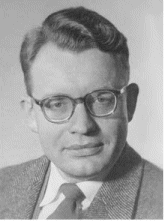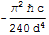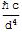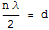# The Casimir EffectIF you take a charged parallel plate capacitor and increase the distance between its plates, you will find that you have to have to do work to overcome the attractive Coulomb force between the plates. If the capacitor plates have area A, and a surface charge density sigma, the amount of work you have to do to increase the plate separation by an amount delta d is:If the capacitor is uncharged, you would expect that no work is required to increase the plate separation (you may assume the ideal case of massless, perfectly conducting plates). However, in 1948, Hendrik Casimir realized, amazingly enough, that is not true.

Casimir showed that an attractive (non-gravitational) quantum force exists between two uncharged perfectly conducting parallel plates, and this result is called the Casimir Effect. For a plate separation of d, the Casimir force per unit area is given by:The exact derivation of the Casimir force equation above is a little involved, but a heuristic argument, plus or minus a multiplicative constant, is possible via dimensional analysis.The first step in any dimensional analysis argument is to specify on what physical quantities the object in question should depend. As the Casimir force is a quantum effect, it must depend on Planck's constant h.
It should also depend on d, the plate separation. If the force is mediated by particles that travel at c (as is the case of the electromagnetic force and photons), then it should also depend on c.So we want Force/area = F(h,d,c) as the required functional dependence, the exact form of which we must determine.
The only combination of h [J T], d [L] and c [L/T2] that has units of Force/area is:To get the minus sign and hence show the Casimir force is attractive, we have 2 choices. One is to do the full blown quantum field theory calculation. The second is note that while classically, the vacuum consists of empty space, in quantum theory, due to the Heisenberg time-energy uncertainty principle, vacuum fluctations are allowed, and the vacuum is anything but empty.In quantum theory, the vacuum is filled with virtual particles, and in particular, virtual photons. It is these virtual photons that impart momentum to the plates when they are reflected from them. Note that in between the plates, only a countable infinity (n=1,2,3,...) of virtual photon frequencies are allowed due to the standing wave condition:On the other hand, outside the plates, there is no such constraint, and an aleph nought infinity of frequencies are allowed. So, the pressure on the outside of the plates must be greater than on the inside by virtue of the larger number of frequencies and virtual photons, and we can conclude the plates are being squeezed together...i.e., the force is attractive, which gives the minus sign.